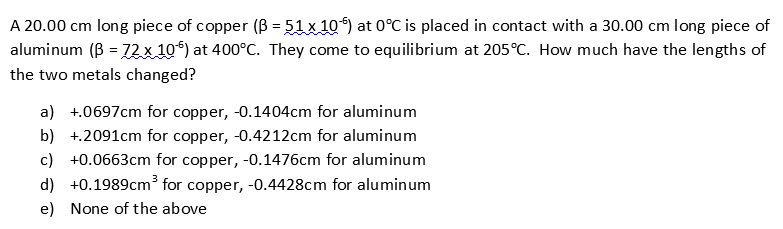A 20.00 cm long piece of copper (B 51 x 10) at 0°C is placed in contact with a 30.00 cmaluminum (B= 72 x 10) at 400°C. They come to equilibrium at 205°C. How much have the lengths oflong piece of-the two metals changed?a) 0697cm for copper, -0.1404cm for aluminumb) 2091cm for copper, -0.4212cm for aluminumc) +0.0663cm for copper, -0.1476cm for aluminum3d) 0.1989cm* for copper, -0.4428cm for aluminume) None of the above

Questionhelp_outlineImage TranscriptioncloseA 20.00 cm long piece of copper (B 51 x 10) at 0°C is placed in contact with a 30.00 cm aluminum (B= 72 x 10) at 400°C. They come to equilibrium at 205°C. How much have the lengths of long piece of - the two metals changed? a) 0697cm for copper, -0.1404cm for aluminum b) 2091cm for copper, -0.4212cm for aluminum c) +0.0663cm for copper, -0.1476cm for aluminum 3 d) 0.1989cm* for copper, -0.4428cm for aluminum e) None of the above fullscreen
Step 1

Given information:

Initial length of copper (Lc) = 20 cm = 0.2 m

Thermal expansion coefficient of copper (βc) = 51 × 10-6

Initial length of aluminium (Lal) = 30 cm = 0.3 m

Thermal expansion coefficient of aluminium (βal) = 72 × 10-6

Initial temperature of copper (TC(i)) = 00 C

Initial temperature of aluminium (TAL(i)) = 4000 C

Final temperature of both the metals (TC(f)) =(TAL(f)) = 2050 C

Step 2

The change in length for any change in temperature for a metal is given by the equation:

ΔL = L×ΔT×β

Where “L” is the initial length,

“ΔT” is change in temperature i.e. ΔT = (Tf - Ti),

“β” is coefficient of thermal expansion.

So, change in length for copper is given by substituting the corresponding value of copper:

ΔL(copper) = 0.2 × (205 - 0) × (51 × 10-6) = 0.2091 × 10-2 m = 0.2091 cm

Step 3

Change in length for aluminium is given by substituting the corresponding value of aluminium:

ΔL(aluminium) = 0.3 × (205 -400) × (72 × 1...

Want to see the full answer?

See Solution

Want to see this answer and more?

Our solutions are written by experts, many with advanced degrees, and available 24/7

See Solution
Tagged in

Thermodynamics